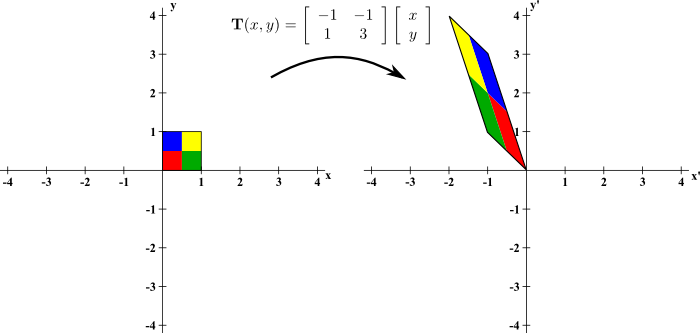# Math Insight

### Image: A stretching and flipping two-dimensional linear transformationThe linear transformation $$\vc{T}(x,y) = \left[\begin{array}{cc}-1 &-1\\ 1 &3\end{array}\right]\left[\begin{array}{c}x\\y\end{array}\right]$$ flips orientation and stretches to increase area by a factor of 2. It maps the unit square $[0,1]\times[0,1]$ into a parallelogram of area 2. The change in orientation is illustrated by the opposite ordering of colors as one moves counterclockwise around the perimeter of the parallelogram as compared to the square. These properties are reflected by the fact that the determinant of the associated matrix is $-2$.

Image file: linear_transformation_2d_m1_m1_1_3.png

Source image file: linear_transformation_2d_m1_m1_1_3.svg
Source image type: Inkscape SVG